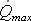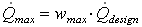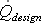# HOMER Pro 3.14Type: Intermediate Variable Units: m3/s Symbol:The maximum flow rate is the maximum allowable flow rate through the hydro turbine. HOMER calculates the maximum flow rate of the hydro turbine using the following equation:where: wmax = the hydro turbine's maximum flow ratio [%]= the hydro turbine's design flow rate [m3/s]

HOMER uses this value to calculate the hydro turbine flow rate in each time step.